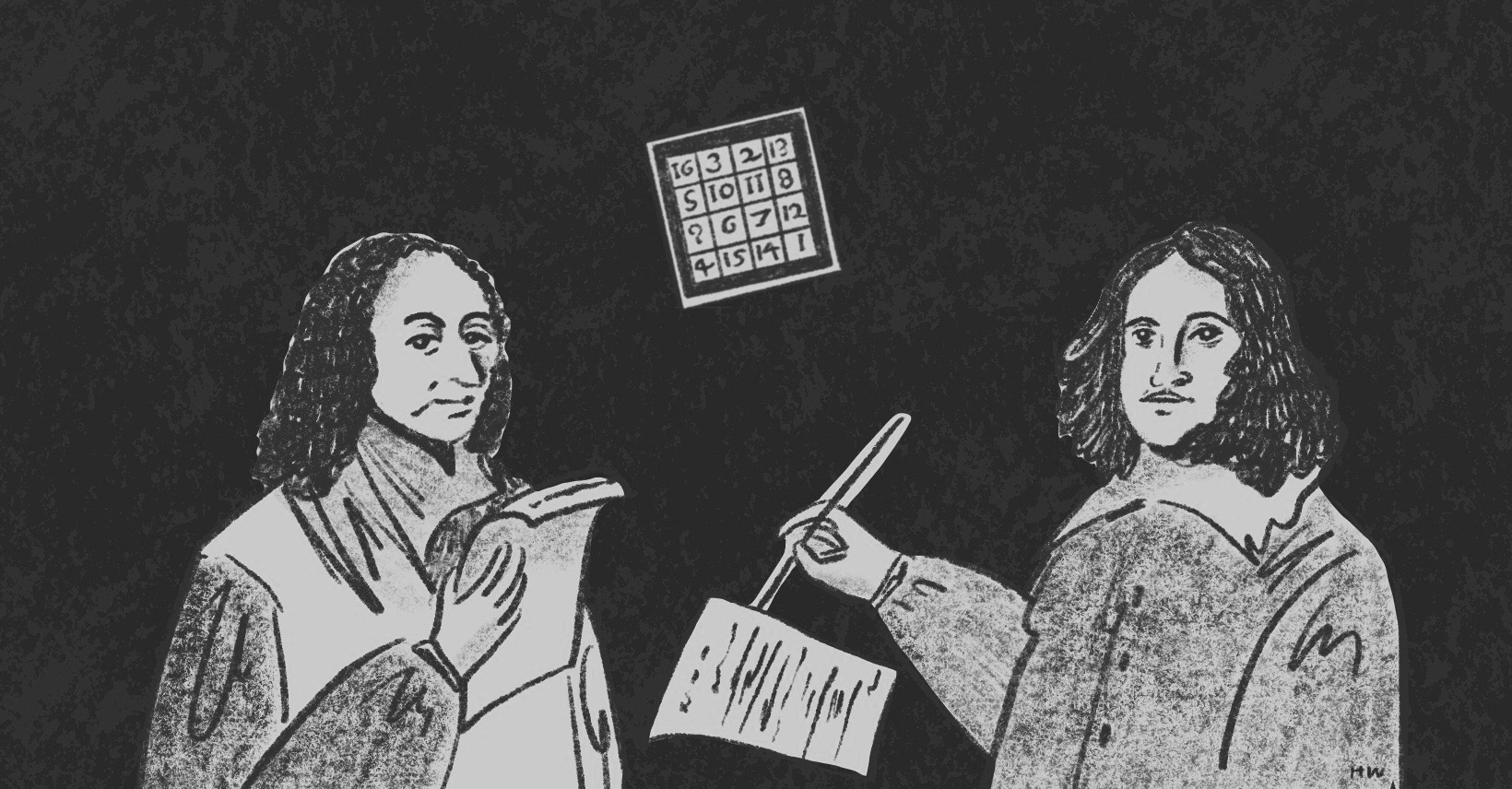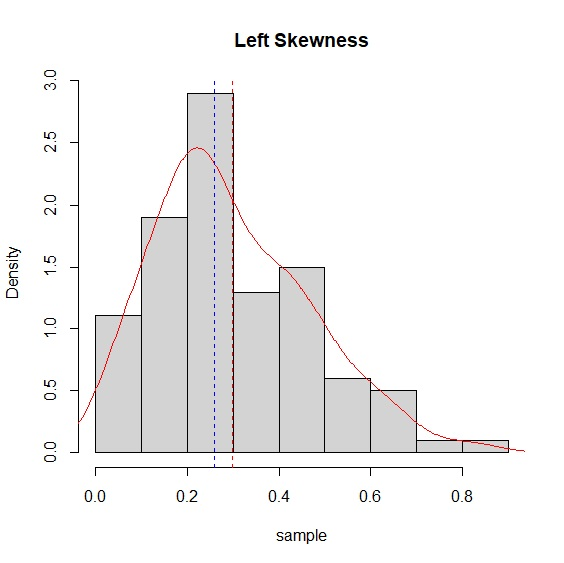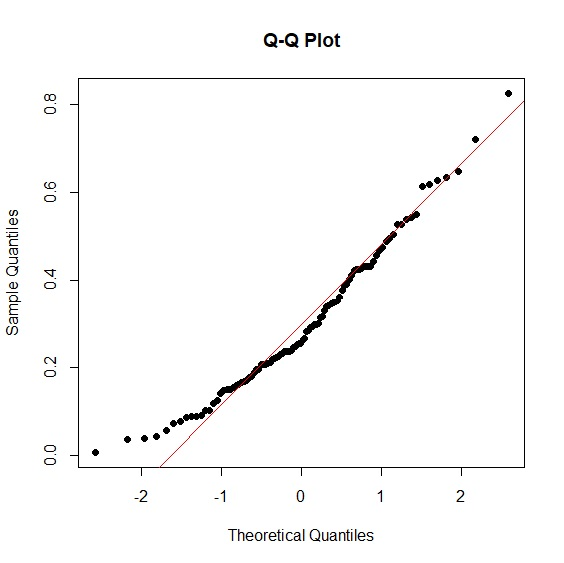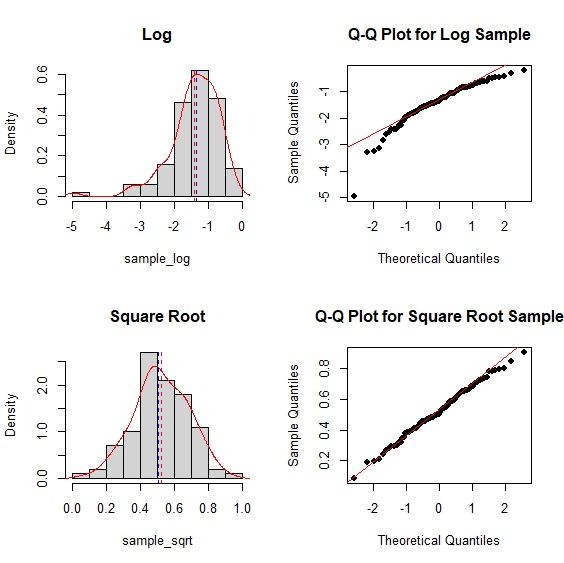×資料管理 統計分析 相關資源 巨人肩膀 語法索引 關於作者

## 簡介

• rbeta() -beta分配
• hist() -直方圖
• lines() -趨勢線
• qqnorm() -常態分配Q-Q圖
• qqline() -常態分配Q-Q線
• wilcox.test() -曼-惠特尼U檢定/魏克生檢定
• kruskal.test() -克拉斯卡－瓦立斯檢定
• cor.test() -相關係數
• RunsTest() -連檢定
• runs.test() -連檢定

## 樣本到底是不是常態分配？

• 樣本取對數
• 樣本開根號
• 無母數統計

 > sample<-rbeta(n=100, shape1=2, shape2=5) > hist(sample, main="Left Skewness", freq=FALSE) > lines(density(sample), col="red") > abline(v=c(mean(sample), median(sample)), col=c("red", "blue"), lty=c(2,2)) > qqnorm(sample, main="Q-Q Plot", pch=16) > qqline(sample, col="red")> shapiro.test(sample) Shapiro-Wilk normality test data: sample W = 0.96423, p-value = 0.008141 

 > par(mfrow=c(2,2)) > hist(sample_log, main="Log", freq=FALSE) > lines(density(sample_log), col="red") > abline(v=c(mean(sample_sqrt), median(sample_sqrt)), col=c("red", "blue"), lty=c(2,2)) > qqnorm(sample_log, main="Q-Q Plot for Log Sample", pch=16) > qqline(sample_log, col="red") > hist(sample_sqrt, main="Square Root", freq=FALSE) > lines(density(sample_sqrt), col="red") > abline(v=c(mean(sample_log), median(sample_log)), col=c("red", "blue"), lty=c(2,2)) > qqnorm(sample_sqrt, main="Q-Q Plot for Square Root Sample", pch=16) > qqline(sample_sqrt, col="red")shapiro檢定p值>.05，證實樣本經過開根號後與常態分配一致。

 > shapiro.test(sample_sqrt) Shapiro-Wilk normality test data: sample_sqrt W = 0.99498, p-value = 0.9747 

## 曼-惠特尼U檢定 Mann-Whitney U Test

$U=min(U_{1},U_{2})$

$U_{1}=n_{1}n_{2}+\frac{n_{1}(n_{1}+1)}{2}-R_{1}, \text{where } R_{1}= \text{變數1的等級總和}$

$U_{2}=n_{1}n_{2}+\frac{n_{2}(n_{2}+1)}{2}-R_{2}, \text{where } R_{2}= \text{變數2的等級總和}$

 > FormosaSurvey<-read.csv("c:/Users/USER/Downloads/formosa_survey.csv", header=T, sep=",") > FormosaSurvey president prime.minister dpp kmt 1 0.578 0.520 0.504 0.336 2 0.598 0.542 0.531 0.333 3 0.593 0.535 0.508 0.354 ⋮ 11 0.555 0.482 0.489 0.278 12 0.546 0.483 0.461 0.280 > wilcox.test(FormosaSurvey$dpp, FormosaSurvey$prime.minister, paired=FALSE) Wilcoxon rank sum exact test data: FormosaSurvey$dpp and FormosaSurvey$prime.minister W = 52, p-value = 0.2657 alternative hypothesis: true location shift is not equal to 0 

## 魏克生等級和檢定 Wilcoxon Rank Sum Test

Frank Wilcoxon在1945年的論文提出等級和檢定(Wilcoxon Rank Sum Test)以及符號等級檢定(Wilcoxon Signed Rank Test)，讓這兩個方法迄今仍以他的名字來命名。等級和檢定其實就是U檢定中的R，R的指令一樣是wilcox.test(paired=FALSE)。

 > wilcox.test(FormosaSurvey$dpp, FormosaSurvey$prime.minister, paired=FALSE) Wilcoxon rank sum test with continuity correction data: FormosaSurvey$dpp and FormosaSurvey$kmt W = 144, p-value = 3.63e-05 alternative hypothesis: true location shift is not equal to 0 

## 魏克生符號等級檢定 Wilcoxon Signed Rank Test

$R=min(R^+, R^-)$

covid-19疫情影響最大的莫過於航空及旅遊業，在邊境管制與隔離的情況下，全球航班大減。台灣儘管疫情相對緩和，但受到其他國家邊境封鎖的情況下，以往出國的熱潮以不復見。departure.csv整理了2019年到2020年桃園機場客運量。我們以魏克生符號等級檢定比較疫情爆發前後離境出國人數的差異。檢定結果p值不僅<.05還<.001顯示疫情的巨大衝擊。 其手動計算可以參考Wilcoxon Sign Step by Step

 > departure<-read.csv("c:/Users/USER/Downloads/departure.csv", header=T, sep=",") > departure year departure 1 2019 2057706 2 2019 1930695 3 2019 2056959 ⋮ 23 2020 40666 24 2020 39025 > wilcox.test(departure~year, paired=TRUE, data=departure) Wilcoxon signed rank exact test data: departure by year V = 77, p-value = 0.0009766 alternative hypothesis: true location shift is not equal to 0 

## 克拉斯卡－瓦立斯檢定 Kruskal-Wallis Test

$K=(N-1)\frac{\sum_{i=1}^g n_{i} (\bar{r_{i}}-\bar{R})^2}{\sum_{i=1}^g \sum_{j=1}^{n_{i}}(r_{ij}-\bar{R})^2}$

 > mullet<-read.csv("c:/Users/USER/Downloads/mullet.csv", header=T, sep=",") > mullet year fishery mullet 1 2011 offshore 221 2 2012 offshore 756 3 2013 offshore 514 ⋮ 29 2019 aquaculture 1985 30 2020 aquaculture 1326 > kruskal.test(mullet~fishery, data=mullet) Kruskal-Wallis rank sum test data: mullet by fishery Kruskal-Wallis chi-squared = 22.764, df = 2, p-value = 1.14e-05 

## 斯皮爾曼與肯道爾等級相關係數 Spearman's $$\rho$$ & Kendall's $$\tau$$

Charles Spearman(1904)賦予相關係數「等級」的概念，迄今仍是衡量兩個變數相關性的無母數指標。一個有趣的插曲是這篇論文第77頁，Spearman引用皮爾森相關係數公式時，犯了一個把平均數當作中位數的明顯錯誤，不過這個小瑕疵一點都不影響這篇論文的重要性。斯皮爾曼等級相關係數的公式與皮爾森相關係數相同，只不過把本來的數值換成等級來計算。在兩個變數等級都不相同的情況下，實際應用中它有個更方便的公式：

$\rho=1-\frac{6 \sum d_{i}^2}{n(n^2-1)}$

$\tau=\frac{協調配對的個數數目-不協調配對的個數數目}{\binom{n}{2}}$

### Pearson's $$r$$

 FormosaSurvey<-read.csv("c:/Users/USER/Downloads/formosa_survey.csv", header=T, sep=",") > attach(FormosaSurvey) > cor.test(president, prime.minister, method=c("pearson")) Pearson's product-moment correlation data: president and prime.minister t = 9.5892, df = 10, p-value = 2.332e-06 alternative hypothesis: true correlation is not equal to 0 95 percent confidence interval: 0.8259683 0.9861258 sample estimates: cor 0.9496924 

### Spearman's $$\rho$$

 > cor.test(president, prime.minister, method=c("spearman")) Spearman's rank correlation rho data: president and prime.minister S = 10, p-value < 2.2e-16 alternative hypothesis: true rho is not equal to 0 sample estimates: rho 0.965035 

### Kendall's $$\tau$$

 > cor.test(president, prime.minister, method=c("kendall")) Kendall's rank correlation tau data: president and prime.minister T = 62, p-value = 5.319e-06 alternative hypothesis: true rho is not equal to 0 sample estimates: tau 0.8787879 

## 連檢定 Wald-Wolfowitz Runs Test

• 情況一：正正反正反正反正正反
• 情況二：正正正反反正正正正反

$\mu=\frac{2N_{+}N_{-}}{N}+1$

$\sigma^2=\frac{2N_{+}N_{-}(2N_{+}N_{-}-N)}{N^2(N-1)}$

 > earthquake<-read.csv("c:/Users/USER/Downloads/earthquake.csv", header=TRUE, sep=",") > str(earthquake) 'data.frame': 365 obs. of 2 variables: $date : chr "1月1日" "1月2日" "1月3日" "1月4日" ...$ earthquake: int 0 0 0 0 0 1 1 1 1 1 ... > library(DescTools) > RunsTest(earthquake$earthquake) Runs Test for Randomness data: earthquake$earthquake z = -2.5022, runs = 101, m = 293, n = 72, p-value = 0.01234 alternative hypothesis: true number of runs is not equal the expected number 

 > earthquake2021<-read.csv("c:/Users/USER/Downloads/earthquake2021.csv", header=TRUE, sep=",") > str(earthquake2021) 'data.frame': 496 obs. of 7 variables: $編號 : chr "小區域有感地震" "小區域有感地震" "小區域有感地震" "小區域有感地震" ...$ 地震時間: chr "2021/1/1 1:42" "2021/1/1 12:57" "2021/1/2 21:26" "2021/1/3 23:09" ... $經度 : num 122 121 122 122 121 ...$ 緯度 : num 24.5 23.6 24.1 24.7 23.8 ... $規模 : num 4.5 3.2 4.8 3.6 3.9 4.3 4.3 4.1 4.1 4 ...$ 深度 : num 65.2 14 25.6 10.3 19.7 13.6 14.1 19.9 12.8 24.2 ... $位置 : chr "宜蘭縣政府南方 22.6 公里 (位於宜蘭縣南澳鄉)(宜蘭縣南澳鄉)" > RunsTest(earthquake2021$規模) Runs Test for Randomness data: earthquake2021$規模 z = -2.1062, runs = 222, m = 277, n = 219, p-value = 0.035194 alternative hypothesis: true number of runs is not equal the expected number sample estimates: median(x) 3.9  連檢定結果共有222個連，規模小於中位數3.9的地震共發生277次，大於中位數3.9的地震共發生219次，p值同樣<.05，所以過去一年來地震發生的規模大小也是隨機。RunsTest()決定$$N_{+}$$、$$N_{-}$$的預設切點是中位數，我們也可以用(x>mean(x))的方法改為平均數。  > RunsTest(earthquake2021$規模>mean(earthquake2021$規模)) Runs Test for Randomness data: earthquake2021$規模 > mean(earthquake2021$規模) z = -2.1062, runs = 222, m = 277, n = 219, p-value = 0.03519 alternative hypothesis: true number of runs is not equal the expected number  randtests套件的runs.test()是另一個可以計算連檢定的指令。與RunsTest()相較，runs.test()會排除那些與中位數數值相同的樣本。例如改以runs.test()排除規模3.9的地震後，樣本從本來的496次縮減為460次，連數縮小為207，不過p值仍然達到顯著水準，可見地震規模也是符合隨機分布。  > library(randtests) > runs.test(earthquake2021$規模) Runs Test data: earthquake2021\$規模 statistic = -2.1964, runs = 207, n1 = 219, n2 = 241, n = 460, p-value = 0.02806 alternative hypothesis: nonrandomness